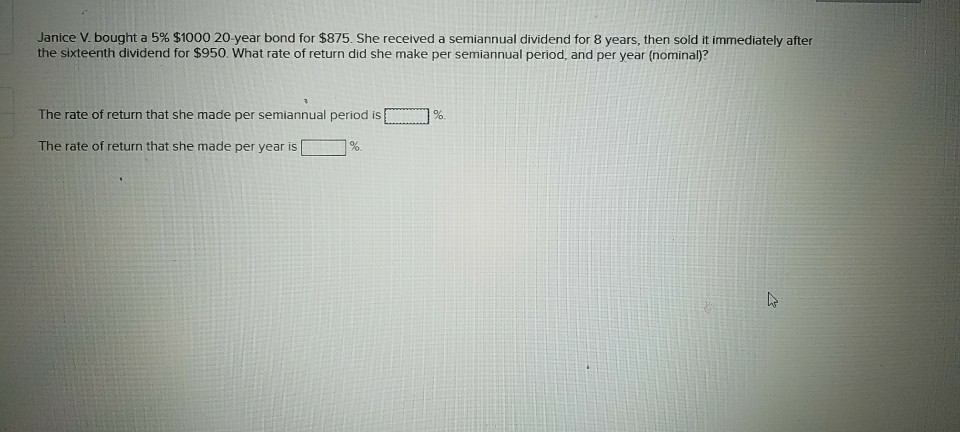# Question & Answer: Janice V. bought a 5% \$1000 20-year bond for \$875. She received a semiannual dividend for 8 years, then sold it immedi…..Janice V. bought a 5% \$1000 20-year bond for \$875. She received a semiannual dividend for 8 years, then sold it immediately after the sixteenth dividend for \$950. What rate of return did she make per semiannual period, and per year (nominal)? The rate of return that she made per semiannual period is %. The rate of return that she made per year is [ ] %.

Don't use plagiarized sources. Get Your Custom Essay on
Question & Answer: Janice V. bought a 5% \$1000 20-year bond for \$875. She received a semiannual dividend for 8 years, then sold it immedi…..
GET AN ESSAY WRITTEN FOR YOU FROM AS LOW AS \$13/PAGE

For computing the semi annual rate of return, we need to use trail and error technique………….

(1) so let us assume 4% return semi annually.

CF = Cash flow = 1000 * 5% * 1/2 = 25

DF = Discounting factor ……….For 1 – 16 periods we take sum of all discounting factors. But for 16th period maturity value only that years DF is taken.

PV = CF * DF

 Int Periods CF DF PV 1 to 16 25 11.6523 291.3074 16 950 0.533908 507.2128 798.5202

This present value of 798.52 is less than the 875. So let us decrease the discount rate to 3%

 Int Periods CF DF PV 1 to 16 25 12.5611 314.0276 16 950 0.623167 592.0086 906.0361

Thus the required rate of return is between 3% and 4%. We use simple interpolation to find the rate which equals 875.

 3 906 x 875 4 799 (x -3) / (4 -3) = (875 – 906) / (799 – 906) x – 3 = – 31 / – 107 x – 3 = 0.29 X = 3.29

a) Thus semi annual rate of return = 3.29%

b) Effective annual rate = ( 1.0329)2 – 1 = 0.0669 or 6.69%